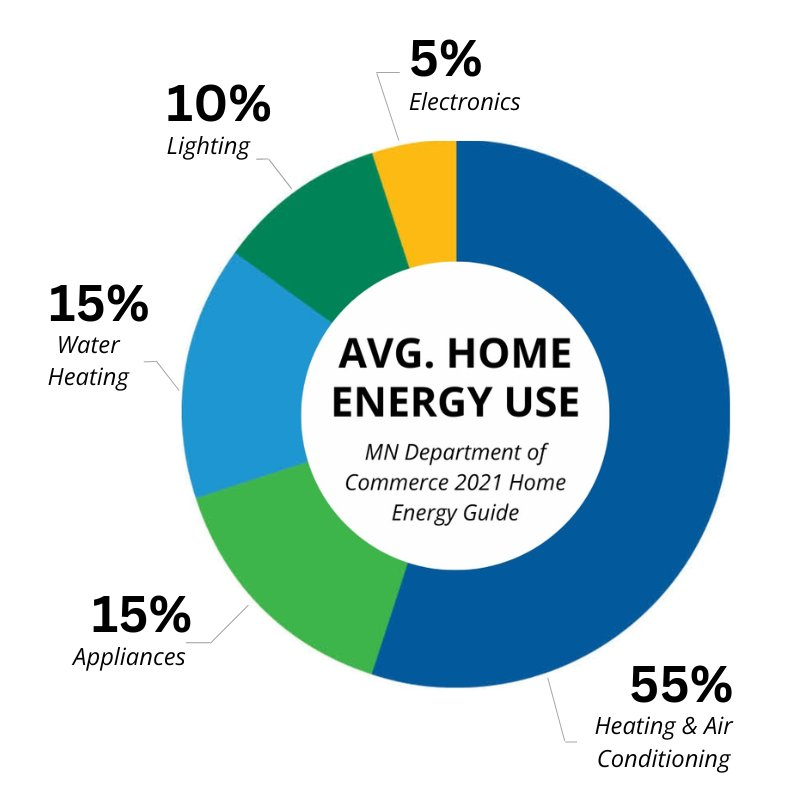# Estimating Electricity Use & Cost

Step 1: Your electric bill is determined by the number of kilowatt-hours (kWh) used during a billing period. For 2022, the average kWh cost was 12.92¢.

Step 2: Wattage determines electrical use per hour and can be found on the serial plate of the appliance. Electric load may also be expressed in volts and amps, rather than watts. If so, multiply volts times amperes to determine the wattage.

120 volts x 12.1 amps = 1,452 watts.

Step 3: Use the formula shown in the following example to estimate use and cost. A light uses 100 watts and is left on for 15 hours. How many kWh are used and what does it cost you?

kWh used = (100 watts x 15 hours) ÷ 1,000 watts = 1.5 kWh. Your cost = 1.5 kWh x 12.8¢ = 19.38¢.